# Adding Whole Numbers & Fractions

An error occurred trying to load this video.

Try refreshing the page, or contact customer support.

Coming up next: Order of Operations with Fractions

### You're on a roll. Keep up the good work!

Replay
Your next lesson will play in 10 seconds
• 0:03 Visualize
• 0:24 Whole Numbers & Proper…
• 1:22 Whole Numbers &…
• 2:02 Making a Whole Number…
• 2:21 Making Common Denominators
• 3:14 Lesson Summary
Save Save

Want to watch this again later?

Timeline
Autoplay
Autoplay
Speed Speed

#### Recommended Lessons and Courses for You

Lesson Transcript
Instructor
Nola Bridgens

Nola has taught elementary school and tutored for four years. She has a bachelor's degree in Elementary Education, a master's degree in Marketing, and is a certified teacher.

Expert Contributor
Donna Blackney

Donna is an adjunct professor at Drexel University with 12 years of teaching experience. She teaches lecture, recitation, and lab courses for general and analytical chemistry.

Although whole numbers and fractions do not look the same, it is still possible to add these two types of numbers together. In this lesson, you will learn how to add whole numbers and fractions.

## Visualize

A whole number is a number without a fractional part; in other words, a number that represents a whole.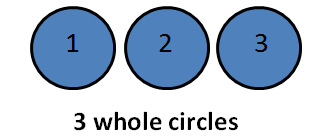A fraction is a number that represents a part of a whole. The numerator is the top number of a fraction that tells how many pieces you have, and the denominator is the bottom number of a fraction that tells how many total pieces the whole is broken into.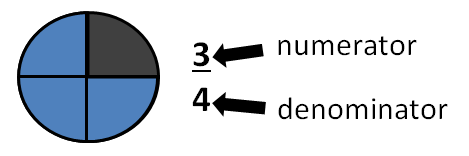## Whole Numbers and Proper Fractions

Sometimes it is necessary to add numbers that are not in the same format. Let's take a look how to do that. Your baby brother ate 2/3 of a piece of pizza, and you ate 3 pieces. How many pieces did you eat all together?

The question is asking you to add 2/3, a fraction, and 3, a whole number. Look at the image to visualize how much pizza you both ate.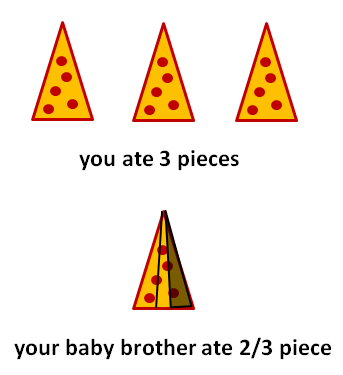If you have a whole number and a proper fraction, you can simply combine the two elements. A proper fraction is a fraction that is less than one and the numerator is smaller than the denominator.

For our pizza example, the fraction 2/3 is proper because it is less than one and the numerator (2) is smaller than the denominator (3). We can simply combine 3 and 2/3 to make 3 2/3. You and your baby brother ate 3 2/3 pieces of pizza.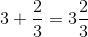You can only use this method with proper fractions. Adding whole numbers and improper fractions requires different steps.

## Whole Numbers and Improper Fractions

An improper fraction is a fraction that represents more than one whole, and the numerator is larger than the denominator. For example, you ate 3 pieces of pizza, and your big sister ate 4/3 pieces of pizza. How much did you eat together?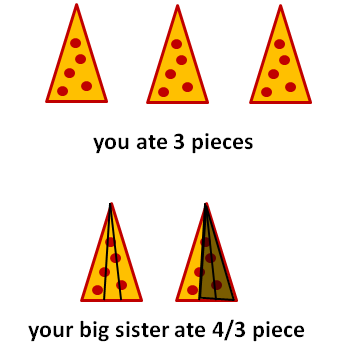You ate 3 pieces, a whole number, and your sister ate 4/3 pieces, an improper fraction. 4/3 represents more than 1 whole, and the numerator (4) is larger than the denominator (3).

1. Turn the whole number into a fraction
2. Give the fractions a common denominator

Let's looks at each of these steps:

To unlock this lesson you must be a Study.com Member.

## Whole Numbers & Fractions Activity

Use a piece of paper to cut out two 4 inch by 4 inch squares. One 4 inch by 4 inch square is equal to one (a whole number). Use a pen or marker to draw one horizontal and one vertical line inside the squares, creating four equal squares. Next, cut out four 1 inch by 1 inch squares. Each square is equal to one-quarter (1/4). Repeat this process until there are twelve 1 inch by 1 inch squares. Using the 4 inch by 4 inch squares as a guide, create the following fractions using the 1 inch by 1 inch squares:

1/4

2/4

4/4 (note that four quarters equals 1 whole)

5/4

7/4

Using the 4 inch by 4 inch squares as a guide, practice adding fractions by combining the following combinations of 1 inch by 1 inch squares that represents fractions with common denominators:

1/4 + 2/4 (3/4 of the 4 inch by 4 inch square are filled after this addition)

1/4 + 3/4 (Notice that the square is completed so 4/4 = 1)

Now, let's look at the addition of improper fractions:

5/4 + 1/4 = 6/4 (There is one complete square (4/4) and two one-quarter squares represented. This equals 1 1/2.)

### Register to view this lesson

Are you a student or a teacher?

#### See for yourself why 30 million people use Study.com

##### Become a Study.com member and start learning now.
Back
What teachers are saying about Study.com

### Earning College Credit

Did you know… We have over 200 college courses that prepare you to earn credit by exam that is accepted by over 1,500 colleges and universities. You can test out of the first two years of college and save thousands off your degree. Anyone can earn credit-by-exam regardless of age or education level.